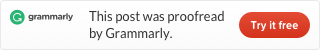Categories

# LeetCode 844. Backspace String Compare (Java)

Given two strings `S` and `T`, return if they are equal when both are typed into empty text editors. `#`means a backspace character.

Example 1:

```Input: S = "ab#c", T = "ad#c"
Output: true
Explanation: Both S and T become "ac".
```

Example 2:

```Input: S = "ab##", T = "c#d#"
Output: true
Explanation: Both S and T become "".
```

Example 3:

```Input: S = "a##c", T = "#a#c"
Output: true
Explanation: Both S and T become "c".
```

Example 4:

```Input: S = "a#c", T = "b"
Output: false
Explanation: S becomes "c" while T becomes "b".
```

Note:

1. `1 <= S.length <= 200`
2. `1 <= T.length <= 200`
3. `S` and `T` only contain lowercase letters and `'#'` characters.

• Can you solve it in `O(N)` time and `O(1)` space?

Given two strings `S` and `T`, return if they are equal when both are typed into empty text editors. `#`means a backspace character.

Example 1:

```Input: S = "ab#c", T = "ad#c"
Output: true
Explanation: Both S and T become "ac".
```

Example 2:

```Input: S = "ab##", T = "c#d#"
Output: true
Explanation: Both S and T become "".
```

Example 3:

```Input: S = "a##c", T = "#a#c"
Output: true
Explanation: Both S and T become "c".
```

Example 4:

```Input: S = "a#c", T = "b"
Output: false
Explanation: S becomes "c" while T becomes "b".
```

Note:

1. `1 <= S.length <= 200`
2. `1 <= T.length <= 200`
3. `S` and `T` only contain lowercase letters and `'#'` characters.

• Can you solve it in `O(N)` time and `O(1)` space?

Given two strings `S` and `T`, return if they are equal when both are typed into empty text editors. `#`means a backspace character.

Example 1:

```Input: S = "ab#c", T = "ad#c"
Output: true
Explanation: Both S and T become "ac".
```

Example 2:

```Input: S = "ab##", T = "c#d#"
Output: true
Explanation: Both S and T become "".
```

Example 3:

```Input: S = "a##c", T = "#a#c"
Output: true
Explanation: Both S and T become "c".
```

Example 4:

```Input: S = "a#c", T = "b"
Output: false
Explanation: S becomes "c" while T becomes "b".
```

Note:

1. `1 <= S.length <= 200`
2. `1 <= T.length <= 200`
3. `S` and `T` only contain lowercase letters and `'#'` characters.

• Can you solve it in `O(N)` time and `O(1)` space?